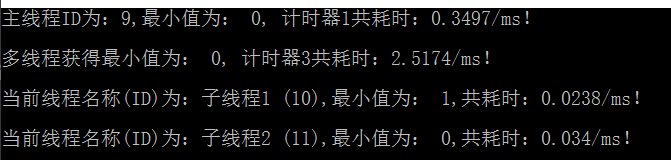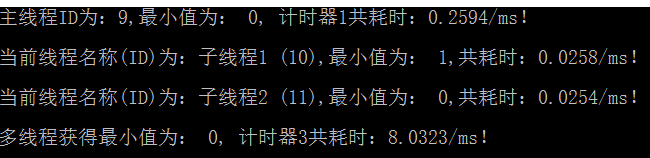C# 多线程，记录每个线程运行时间

using System;
using System.Collections.Generic;
using System.Linq;
using System.Diagnostics;

``````namespace Multithread_PSO
{
class Program
{
//定义锁
private static readonly object locker = new object();
static void Main(string[] args)
{
//元素个数
int EleCounts = 100000;
//待处理数组
int[] MyArr = new int[EleCounts];
//前半段数组
int[] TempArr1 = new int[EleCounts / 2];
//后半段数组
int[] TempArr2 = new int[EleCounts / 2];

for (int i = 0; i < EleCounts; i++)
{
int iSeed = DateTime.Now.Millisecond;
Random rd = new Random(iSeed+i);
//随机给数组赋值
MyArr[i] = rd.Next(EleCounts);
//将数组拆分为两个大小相等的数组
if(i<EleCounts/2)
{
TempArr1[i] = MyArr[i];
}
else
{
TempArr2[i - EleCounts / 2] = MyArr[i];
}
}
//主线程
FindMinElement(MyArr);
//新建两个线程th1，th2
th1.Name = "子线程1";
th2.Name = "子线程2";

Stopwatch sw = new Stopwatch();
sw.Start();
th1.Start(TempArr1);
//th1.Join();
th2.Start(TempArr2);
//th2.Join();
sw.Stop();
TimeSpan ts2 = sw.Elapsed;
Console.WriteLine("多线程获得最小值为： {0}, 计时器3共耗时：{1}/ms！\n", MinValue, ts2.TotalMilliseconds);

}
public static void thFindMinElement(object DivMyArr)
{
Stopwatch sw = new Stopwatch();
sw.Start();
int[] MyArr = (int[])DivMyArr;
if (IsFirstRun)
{
MinValue = MyArr;
IsFirstRun = false;
}

for (int i = 1; i < MyArr.Length; i++)
{
if (MinValue > MyArr[i])
MinValue = MyArr[i];
}
sw.Stop();
TimeSpan ts2 = sw.Elapsed;
}
public static void FindMinElement(int[] DivMyArr)
{
Stopwatch sw = new Stopwatch();
sw.Start();
int[] MyArr = DivMyArr;
MinValue = MyArr;
for (int i = 1; i < MyArr.Length; i++)
{
if (MinValue > MyArr[i])
MinValue = MyArr[i];
}
sw.Stop();
TimeSpan ts2 = sw.Elapsed;
Console.WriteLine("主线程ID为：{0},最小值为： {1}, 共耗时：{2}/ms！\n", ThNumber, MinValue, ts2.TotalMilliseconds);
}

private static int minValue;
private static bool IsFirstRun = true;
private static int MinValue
{
get
{
lock (locker)
{
return minValue;
}
}
set { minValue = value; }
}
}
``````

}2个回答